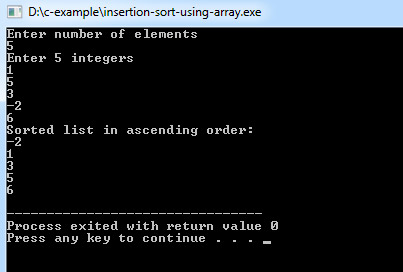# C Program to Insertion Sort Using Array

This C program will show you how to short numbers at the time of Insertion.

This code implements insertion sort algorithm to arrange numbers of an array in ascending order. With a little modification it will arrange numbers in descending order.

Example:
``````/* insertion sort ascending order */
#include <stdio.h>

int main()
{
int n, array, c, d, t;

printf("Enter number of elements\n");
scanf("%d", &n);

printf("Enter %d integers\n", n);

for (c = 0; c < n; c++) {
scanf("%d", &array[c]);
}

for (c = 1 ; c <= n - 1; c++) {
d = c;
while ( d > 0 && array[d] < array[d-1]) {
t          = array[d];
array[d]   = array[d-1];
array[d-1] = t;
d--;
}
}

printf("Sorted list in ascending order:\n");

for (c = 0; c <= n - 1; c++) {
printf("%d\n", array[c]);
}

return 0;
}``````
Program Output:### Here are few other related articles for you to read: